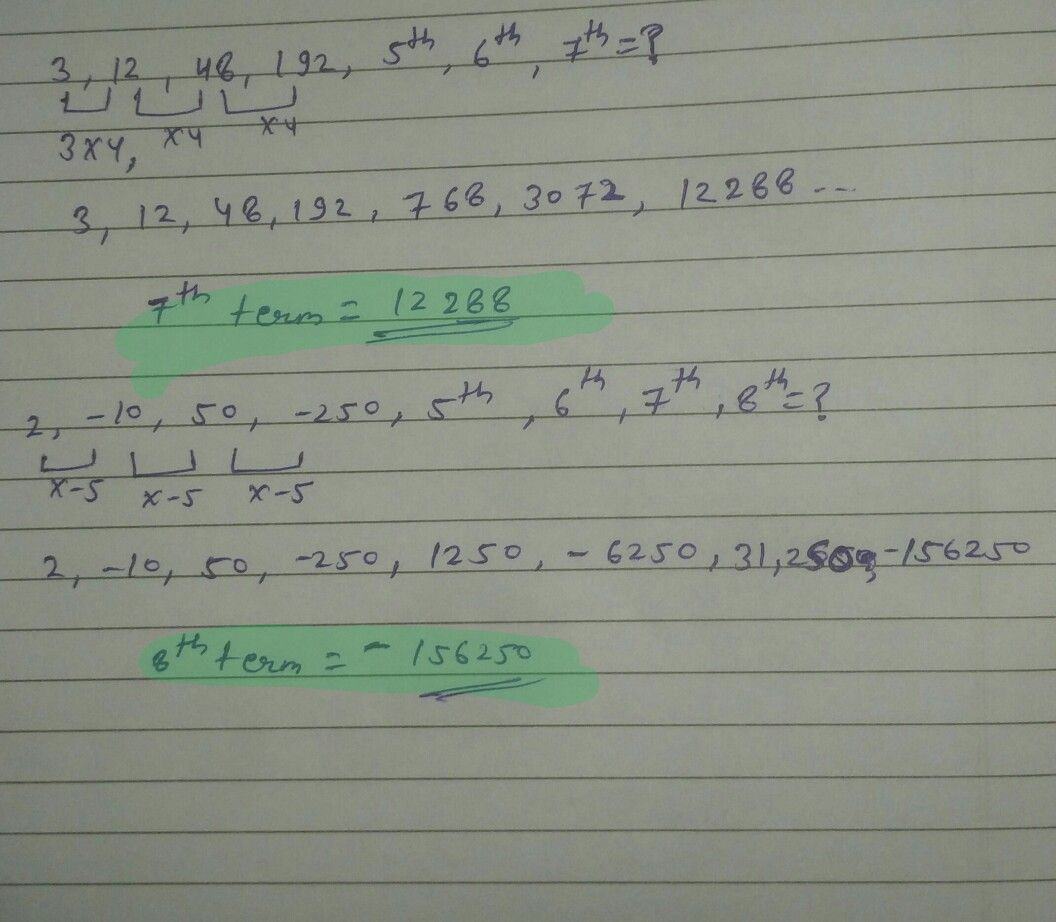Symbol
Problem$192$ $3$ What is the 7th term of the geometric sequence $3$ $12,48,$ $192$ What $1s$ the $8th$ term of the geometric sequence $2,-10,50$ $-250,...7$